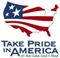Scientific Investigations Report 2008–5025

U.S. GEOLOGICAL SURVEY
Scientific Investigations Report 2008–5025

# Logistic Regression Results

Based on the relations between the explanatory variables and the measured elevated nitrate concentrations, various logistic regression models were developed to estimate the probability that ground water will exceed a nitrate concentration of 2 mg/L. All possible combinations of the explanatory variables were evaluated to develop the models. The performance of these models was evaluated using the log-likelihood ratio, McFadden’s rho-squared, the p-values for each variable included in the models, and the percentage of correct responses.

The two best performing models—one with and one without hydrogeomorphic regions—for estimating the probability of nitrate concentrations exceeding 2 mg/L were identified. Models with and without hydrogeomorphic regions were developed because of the possibility that different relations between explanatory and response variables may occur in each of the hydrogeomorphic regions. This allowed a comparison between the model that included the hydrogeomorphic regions with the one that did not to determine if inclusion of the hydrogeomorphic regions significantly improved model performance.

Well depth, average annual precipitation, percentage of agricultural land within a 4-km buffer around the well, population density, and soil drainage were the significant explanatory variables in both of the best performing models (table 2). Overall performance of both models was good, with the chi-squared p-value calculated from the log-likelihood ratio of the entire model less than 0.001, McFadden’s rho-squared of about 0.19, and the percentage of correct responses greater than 81 percent (table 3). The two models performed equally well, with identical percentages of correct responses.

The positive and negative signs of the model coefficients were as expected. Well depth showed a negative correlation with elevated nitrate concentrations as nitrate concentrations usually decrease with depth below land surface. The relation between percentage of agricultural land use near a well and elevated nitrate concentrations was positive. This indicates that agricultural fertilizer is a large source of nitrate to ground water. The relation between population density and elevated nitrate concentrations also was positive, which is likely the result of residential fertilizer usage and septic system inputs. The relation between elevated nitrate concentrations and soil drainage was negative indicating that the probability of elevated nitrate concentrations increases beneath soils that are well drained. Soils that are poorly drained may have an increased likelihood of fostering denitrifying conditions, thereby decreasing the amount of nitrate available to leach into the ground water. The relation between elevated nitrate concentrations and precipitation also was negative, which is likely due to the larger percentage of elevated nitrate concentrations in eastern Washington, which receives less precipitation than western Washington.

Alternative measures of model performance were generated regressing the measured and estimated probabilities of elevated nitrate concentrations from wells included in the calibration dataset (fig. 7). Nitrate concentrations from the calibration wells were converted to a binary classification of 0 for nitrate concentrations less than 2 mg/L and 1 for those greater than 2 mg/L. This binary conversion allowed the percentage of actual detections to be calculated and compared to the estimated probabilities for each 10 percent decile. The estimated and measured number of exceedances and nonexceedances were similar for both models with and without hydrogeomorphic regions, as indicated by R2values of 0.9748 and 0.9792, respectively, with no systemic bias as shown by the 1:1 line in figure 7. Pearson’s correlation coefficients and Tolerance and VIF statistics detected no multicollinearity for the explanatory variables included in the models. Pearson’s correlation coefficients were all less than 0.5, tolerance was greater than 0.67, and VIF less than 1.5.

## Validation of Nitrate Models

To validate the models, the estimated probabilities of elevated nitrate concentrations for wells in the validation dataset from USGS and Department of Ecology were computed and compared to the actual probabilities (fig. 8). Nitrate concentrations from the validation wells were converted to a binary classification of 0 for nitrate concentrations less than 2 mg/L and 1 for concentrations greater than 2 mg/L. This binary conversion allowed the percentage of measured detections to be calculated and compared to the estimated probabilities for each 10 percent decile. The relation between the actual percentage of wells with a nitrate concentration greater than 2 mg/L and the estimated probabilities had an R2 of 0.9061 for the model without hydrogeomorphic regions and 0.9173 for the model with hydrogeomorphic regions. The validation of the model that included hydrogeomorphic regions was not as strong because some regions had few data points. Overall, both logistic regression models tended to underestimate the actual probability for the validation dataset by about 10 percent.U.S. Department of the Interior | U.S. Geological Survey